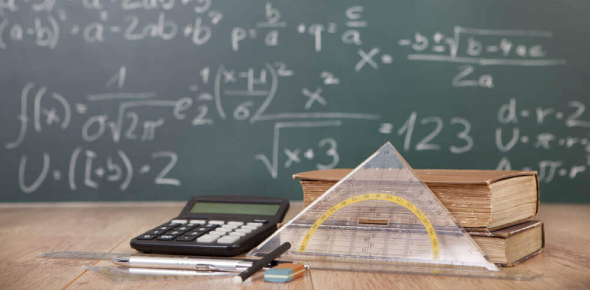# Mathematics Questions: Trivia Test! Quiz

12 Questions | Total Attempts: 651SettingsAre you any good at mathematics, and would you be able to pass this quiz? Math is a complex and often challenging subject to tackle. Mathematics can help you with your everyday problems, such as balancing your budget and paying taxes. Math also helps you with your problem-solving skills. Analytical thinking assists us in looking at problems logically and critically. This quiz will put your knowledge of mathematics to the test.

• 1.
-4(3x+2) expands to become
• A.

12x-2

• B.

-12x+4

• C.

-12x+8

• D.

-12x-8

• 2.
Simplify 7z+3w-4z+3w+6
• A.

15zw

• B.

3z+6w+6

• C.

11z+6w+6

• D.

3z+6w

• 3.
Simplify and combine like terms for 6(3+2y) + 3y=
• 4.
Simplify and combine like terms for 2(8w-4) +10 =
• 5.
Translate the word problems into an equation OR inequality: five less than twice a number
• A.

5 - 2n

• B.

5 - 2 - n

• C.

5 < n

• D.

2n - 5

• 6.
Translate the word problems into an equation OR inequality: the sum of eight and six times a number is 300
• A.

8 - 6x = 300

• B.

8 + 6x = 300

• C.

86x = 300

• D.

6x - 8 = 300

• 7.
Solve the following equation: 4 = 4x - 2 - 5x
• A.

X = 1

• B.

X = 6

• C.

X = -6

• D.

X = -1

• 8.
9x − 7 = −7
• A.

X = 0

• B.

X = 1

• C.

X = 49

• D.

X =-63

• 9.
−9x + 1 = −80
• A.

X = -81

• B.

X = -10

• C.

X = 9

• D.

X = 0

• 10.
Solve the following: 7x+14-3+6x-12x+13+12-2x
Related TopicsBack to top